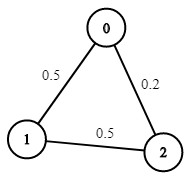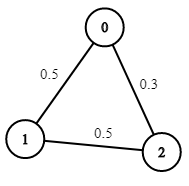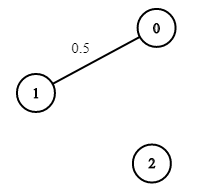Formatted question description: https://leetcode.ca/all/1514.html

# 1514. Path with Maximum Probability (Medium)

You are given an undirected weighted graph of n nodes (0-indexed), represented by an edge list where edges[i] = [a, b] is an undirected edge connecting the nodes a and b with a probability of success of traversing that edge succProb[i].

Given two nodes start and end, find the path with the maximum probability of success to go from start to end and return its success probability.

If there is no path from start to end, return 0. Your answer will be accepted if it differs from the correct answer by at most 1e-5.

Example 1:Input: n = 3, edges = [[0,1],[1,2],[0,2]], succProb = [0.5,0.5,0.2], start = 0, end = 2
Output: 0.25000
Explanation: There are two paths from start to end, one having a probability of success = 0.2 and the other has 0.5 * 0.5 = 0.25.


Example 2:Input: n = 3, edges = [[0,1],[1,2],[0,2]], succProb = [0.5,0.5,0.3], start = 0, end = 2
Output: 0.30000


Example 3:Input: n = 3, edges = [[0,1]], succProb = [0.5], start = 0, end = 2
Output: 0.00000
Explanation: There is no path between 0 and 2.


Constraints:

• 2 <= n <= 10^4
• 0 <= start, end < n
• start != end
• 0 <= a, b < n
• a != b
• 0 <= succProb.length == edges.length <= 2*10^4
• 0 <= succProb[i] <= 1
• There is at most one edge between every two nodes.

Related Topics:
Graph

## Solution 1. Dijkstra

Direct application of Dijkstra algorithm except that usually the cost is the smaller the better, but in this problem the probability is the greater the better.

// OJ: https://leetcode.com/problems/path-with-maximum-probability/

// Time: O(ElogE)
// Space: O(E)
class Solution {
public:
double maxProbability(int n, vector<vector<int>>& edges, vector<double>& succProb, int start, int end) {
vector<vector<pair<int, double>>> G(n);
for (int i = 0; i < edges.size(); ++i) {
auto &e = edges[i];
G[e].emplace_back(e, succProb[i]);
G[e].emplace_back(e, succProb[i]);
}
priority_queue<pair<double, int>> q;// prob, index
q.emplace(1, start);
vector<double> prob(n), seen(n);
prob[start] = 1;
while (q.size()) {
auto p = q.top();
int u = p.second;
q.pop();
if (seen[u]) continue;
seen[u] = 1;
if (u == end) return prob[u];
for (auto &nei : G[u]) {
double v = nei.first, w = nei.second;
if (!seen[v] && prob[v] < prob[u] * w) {
prob[v] = prob[u] * w;
q.emplace(prob[v], v);
}
}
}
return 0;
}
};


Or use multiset

// OJ: https://leetcode.com/problems/path-with-maximum-probability/

// Time: O(ElogE)
// Space: O(E)
class Solution {
public:
double maxProbability(int n, vector<vector<int>>& edges, vector<double>& succProb, int start, int end) {
vector<vector<pair<int, double>>> G(n);
for (int i = 0; i < edges.size(); ++i) {
auto &e = edges[i];
G[e].emplace_back(e, succProb[i]);
G[e].emplace_back(e, succProb[i]);
}
multiset<pair<double, int>> s; // prob, index
unordered_map<int, double> m;
s.emplace(1, start);
while (s.size()) {
auto p = *s.rbegin();
s.erase(prev(s.end()));
int u = p.second;
if (m.count(u)) continue;
m[u] = p.first;
if (u == end) return m[u];
for (auto &[v, w] : G[u]) s.emplace(w * m[u], v);
}
return 0;
}
};


Java

class Solution {
public double maxProbability(int n, int[][] edges, double[] succProb, int start, int end) {
Map<Integer, List<Integer>> edgesMap = new HashMap<Integer, List<Integer>>();
Map<Integer, List<Double>> probabilitiesMap = new HashMap<Integer, List<Double>>();
int edgesCount = edges.length;
for (int i = 0; i < edgesCount; i++) {
int[] edge = edges[i];
double probability = succProb[i];
int node0 = edge, node1 = edge;
List<Integer> edgeList0 = edgesMap.getOrDefault(node0, new ArrayList<Integer>());
List<Integer> edgeList1 = edgesMap.getOrDefault(node1, new ArrayList<Integer>());
List<Double> probabilityList0 = probabilitiesMap.getOrDefault(node0, new ArrayList<Double>());
List<Double> probabilityList1 = probabilitiesMap.getOrDefault(node1, new ArrayList<Double>());
edgesMap.put(node0, edgeList0);
edgesMap.put(node1, edgeList1);
probabilitiesMap.put(node0, probabilityList0);
probabilitiesMap.put(node1, probabilityList1);
}
double[] probabilities = new double[n];
probabilities[start] = 1;
Queue<Integer> queue = new LinkedList<Integer>();
queue.offer(start);
while (!queue.isEmpty()) {
int node = queue.poll();
double probability = probabilities[node];
List<Integer> edgeList = edgesMap.getOrDefault(node, new ArrayList<Integer>());
List<Double> probabilityList = probabilitiesMap.getOrDefault(node, new ArrayList<Double>());
int size = edgeList.size();
for (int i = 0; i < size; i++) {
int nextNode = edgeList.get(i);
double nextProbability = probability * probabilityList.get(i);
if (nextProbability > probabilities[nextNode]) {
probabilities[nextNode] = nextProbability;
queue.offer(nextNode);
}
}
}
return probabilities[end];
}
}